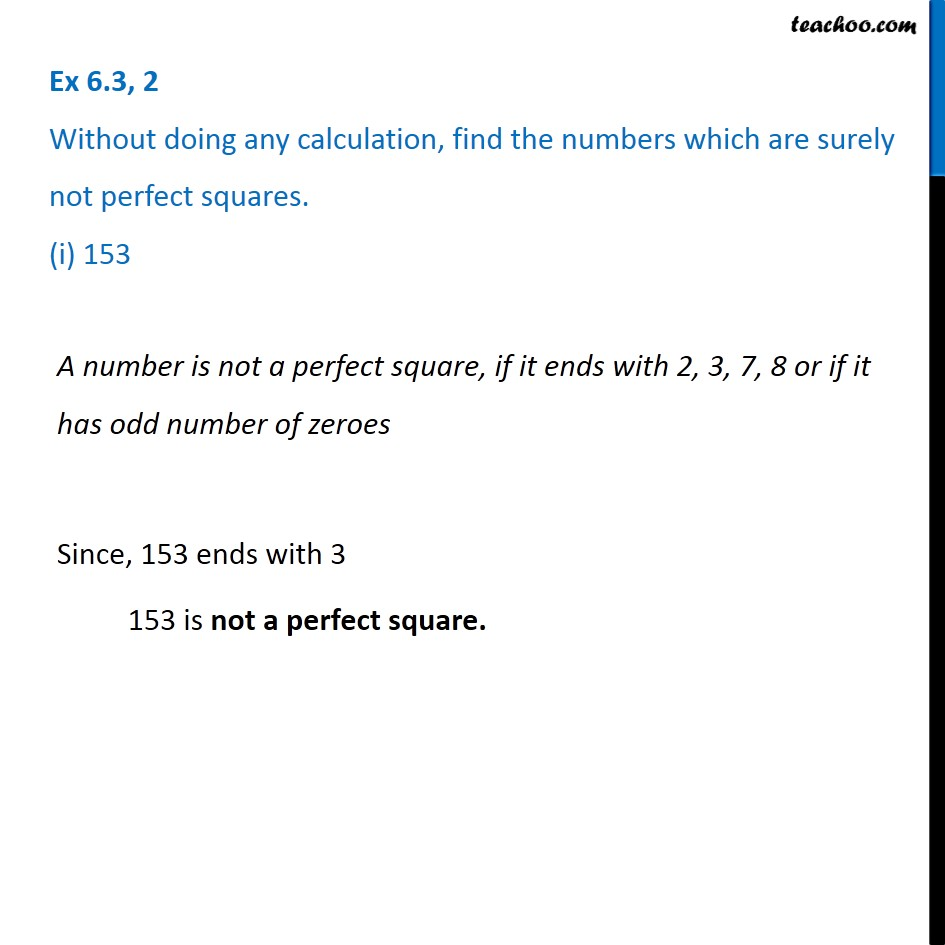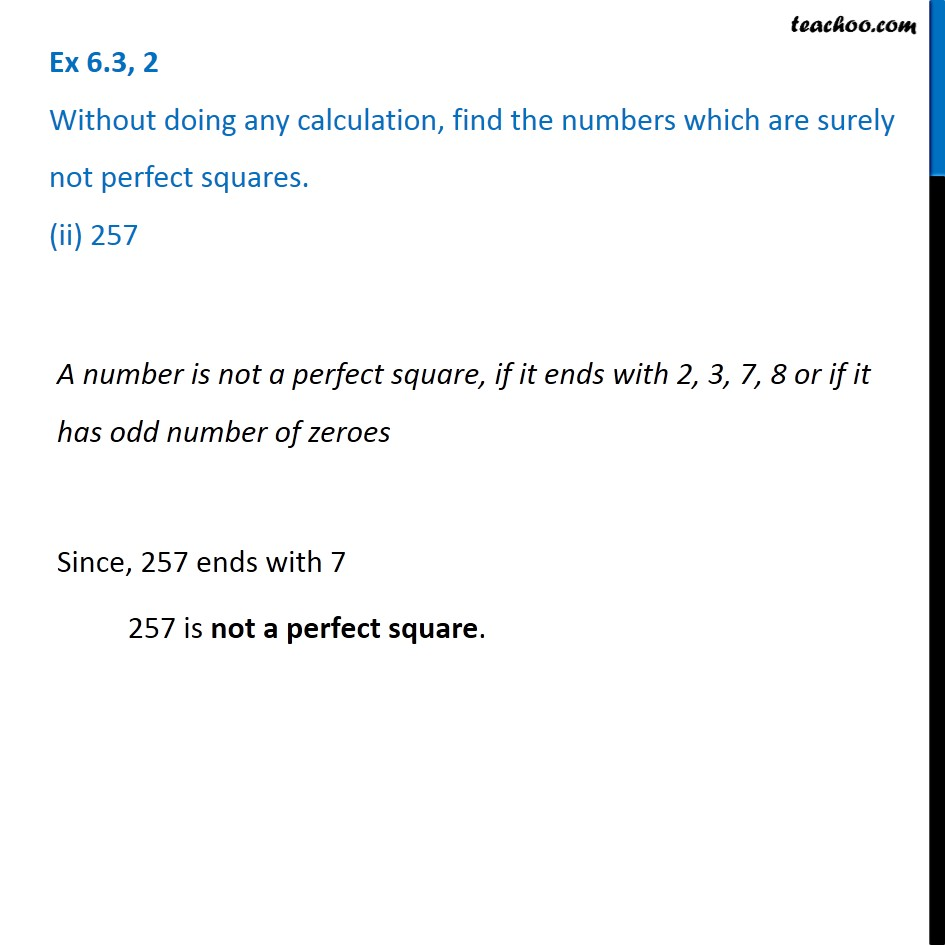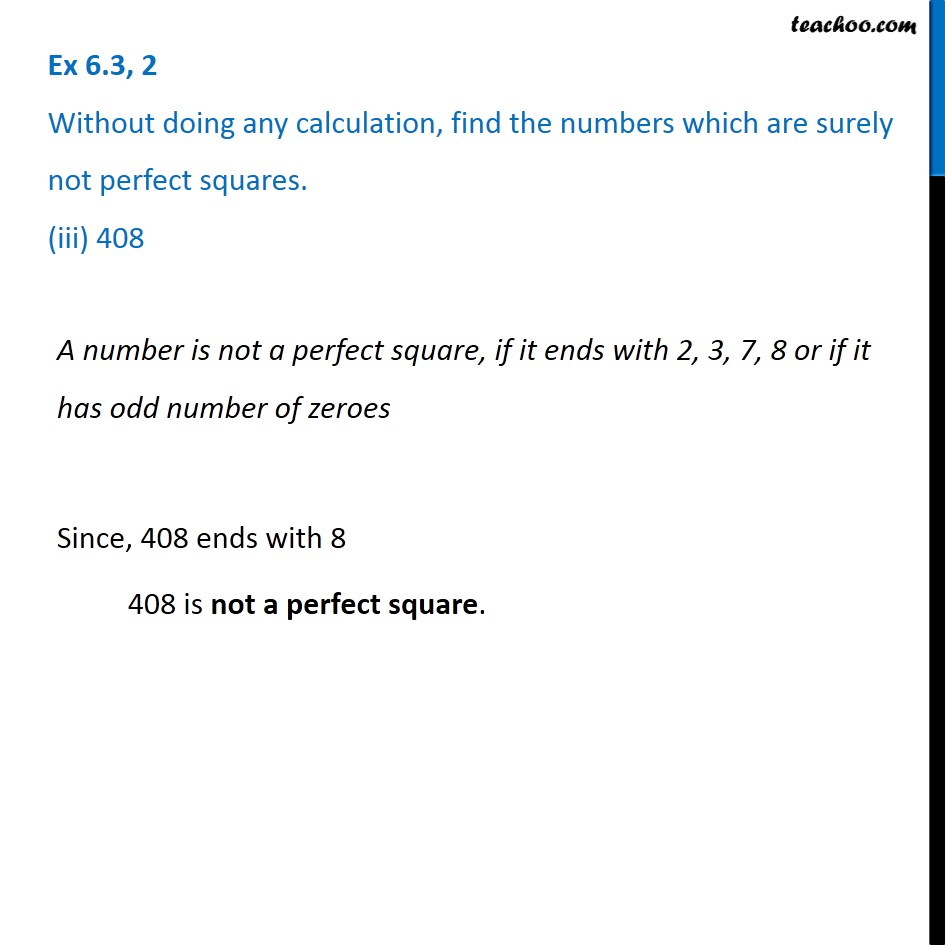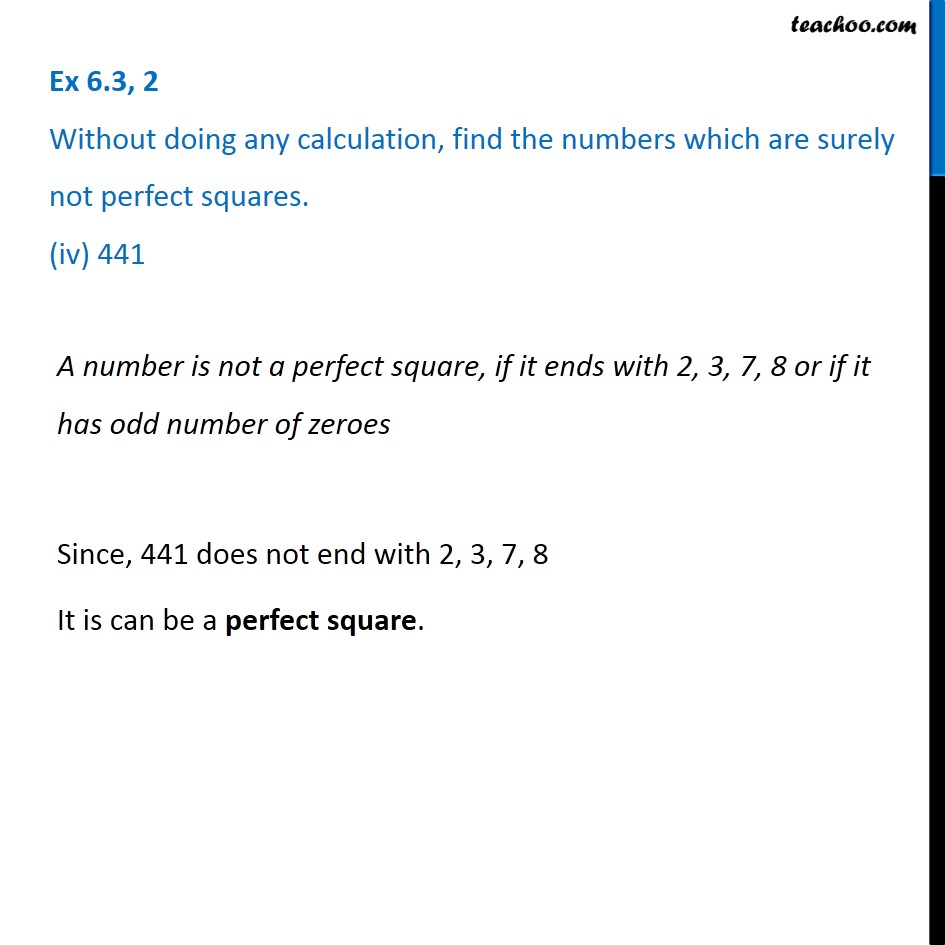Subscribe to our Youtube Channel - https://you.tube/teachoo

1. Chapter 6 Class 8 Squares and Square Roots
2. Serial order wise
3. Ex 6.3

Transcript

Ex 6.3, 2 Without doing any calculation, find the numbers which are surely not perfect squares. (i) 153A number is not a perfect square, if it ends with 2, 3, 7, 8 or if it has odd number of zeroes Since, 153 ends with 3 153 is not a perfect square. Ex 6.3, 2 Without doing any calculation, find the numbers which are surely not perfect squares. (ii) 257A number is not a perfect square, if it ends with 2, 3, 7, 8 or if it has odd number of zeroes Since, 257 ends with 7 257 is not a perfect square. Ex 6.3, 2 Without doing any calculation, find the numbers which are surely not perfect squares. (iii) 408A number is not a perfect square, if it ends with 2, 3, 7, 8 or if it has odd number of zeroes Since, 408 ends with 8 408 is not a perfect square. Ex 6.3, 2 Without doing any calculation, find the numbers which are surely not perfect squares. (iv) 441A number is not a perfect square, if it ends with 2, 3, 7, 8 or if it has odd number of zeroes Since, 441 does not end with 2, 3, 7, 8 It is can be a perfect square.

Ex 6.3